# Probability for compound events#### Everything You Need in One Place

Homework problems? Exam preparation? Trying to grasp a concept or just brushing up the basics? Our extensive help & practice library have got you covered.#### Learn and Practice With Ease

Our proven video lessons ease you through problems quickly, and you get tonnes of friendly practice on questions that trip students up on tests and finals.#### Instant and Unlimited Help

Our personalized learning platform enables you to instantly find the exact walkthrough to your specific type of question. Activate unlimited help now!

0/3
##### Intros
###### Lessons
1. Introduction to Basic Probability for Compound Events:
2. Simple vs. Compound Events in Probability
3. Probability Trees
4. Strategies for Listing Compound Outcomes
0/9
##### Examples
###### Lessons
1. Compound event outcomes and probability trees
Fill in the probability tree and list the outcomes for:
1. Spinning a two-part spinner twice.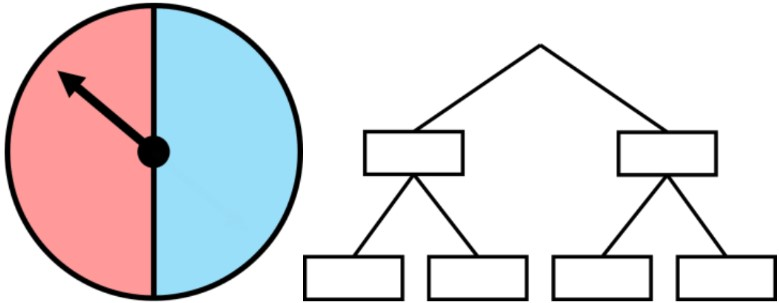2. Rolling two six-sided dice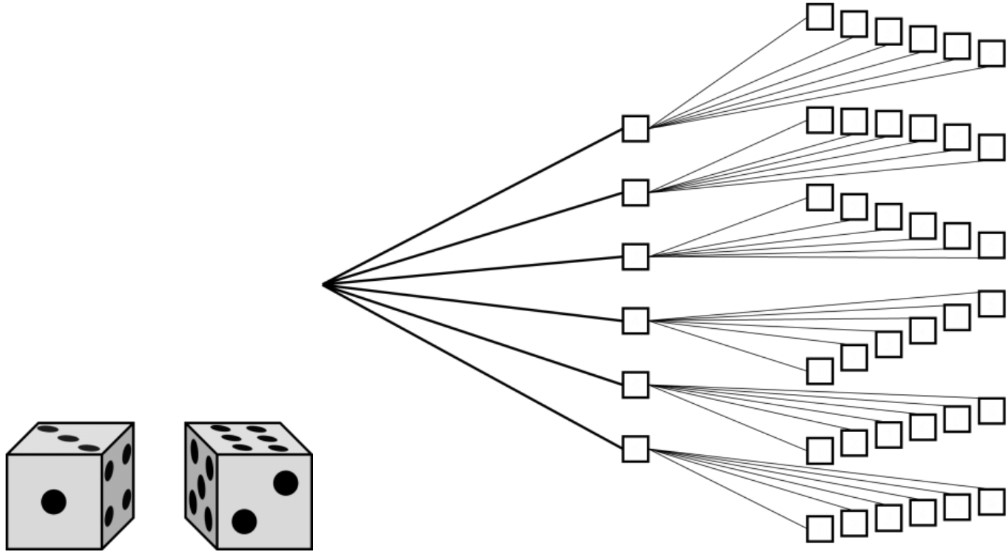2. Probability of compound events
Find the probability for:
1. Using a three-part spinner and: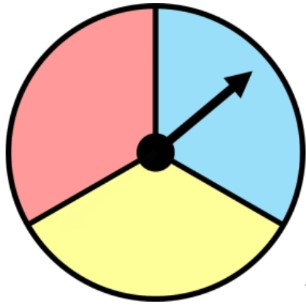1. spinning yellow twice in a row.
2. spinning blue once and red once.
3. spinning yellow first and then red second.
2. Flipping a coin then spinning a two-part spinner and: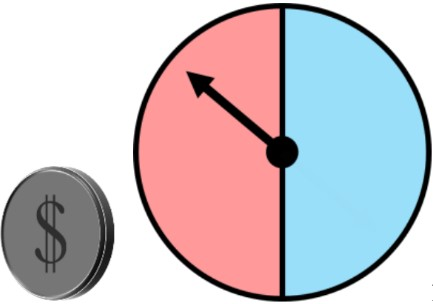1. The coin lands on head, and the spinner lands on red.
2. The coin lands on tail, and the spinner lands on blue.
3. Spinning the arrow on spinner #1 then on spinner #2 and: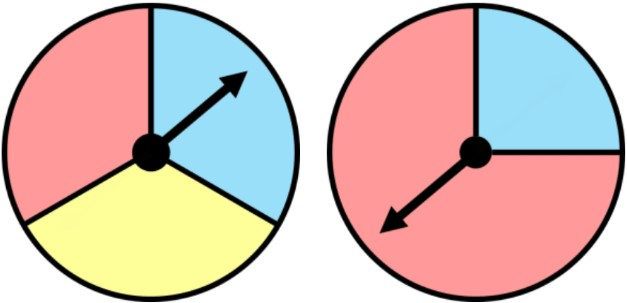1. both spinner #1 and #2 land on red.
2. one spinner lands on red and the other lands on blue.
3. spinner #1 lands on yellow, spinner #2 lands on blue.
3. Probability for compound events: word problem
In a board game, each player needs to roll two dice at the same time during their turn.
1. How many total outcomes are there?
2. There are bonus points for rolling some combinations in this game. What are the probabilities of:
1. Rolling two-6s.
2. Rolling two of the same number.
3. Rolling a sum greater than 8.
4. Rolling a sum less than 4.
3. Which outcome for bonus points is the most likely for this game? Use the answers from the previous part.
4. Using theoretical probability, write the expected results.
1. If you roll the dice 36 times, how many times will you roll one 5 and one 6?
2. If you roll the dice 360 times, how many times will you roll two-6s?
3. If you roll the dice 120 times, how many times will roll two of the same number?
###### Free to Join!
StudyPug is a learning help platform covering math and science from grade 4 all the way to second year university. Our video tutorials, unlimited practice problems, and step-by-step explanations provide you or your child with all the help you need to master concepts. On top of that, it's fun - with achievements, customizable avatars, and awards to keep you motivated.
• #### Easily See Your ProgressWe track the progress you've made on a topic so you know what you've done. From the course view you can easily see what topics have what and the progress you've made on them. Fill the rings to completely master that section or mouse over the icon to see more details.
• #### Make Use of Our Learning Aids###### Practice Accuracy

Get quick access to the topic you're currently learning.

See how well your practice sessions are going over time.

Stay on track with our daily recommendations.

• #### Earn Achievements as You LearnMake the most of your time as you use StudyPug to help you achieve your goals. Earn fun little badges the more you watch, practice, and use our service.
• #### Create and Customize Your AvatarPlay with our fun little avatar builder to create and customize your own avatar on StudyPug. Choose your face, eye colour, hair colour and style, and background. Unlock more options the more you use StudyPug.
###### Topic Notes

In this lesson, we will learn:

• Compound probability is for finding the chances of an event involving two simple probability scenarios
• How to draw a probability tree to show all possible outcomes for a compound event
• How to use an outcome table to show all possible outcomes for a compound event
• How to calculate compound probability fractions

Notes:

• Compound probability (or probability for compound events) is the chances of an event—involving two probability scenarios—happening.
• Some examples of compound situations: flipping 2 coins, rolling 2 dice, spinning the arrow on 2 spinners, or (combinations like) a coin flip and a die roll.

• Showing all possible outcomes for compound events will be different than probability for simple events. A probability tree or outcome table can be used

• A probability tree can be drawn vertically or horizontally. Each level represents each simple event: start at a point and draw branches for all possible outcomes. At the next level, the end of each previous branch will be a new starting point. Draw branches for all possible outcomes for the second simple event.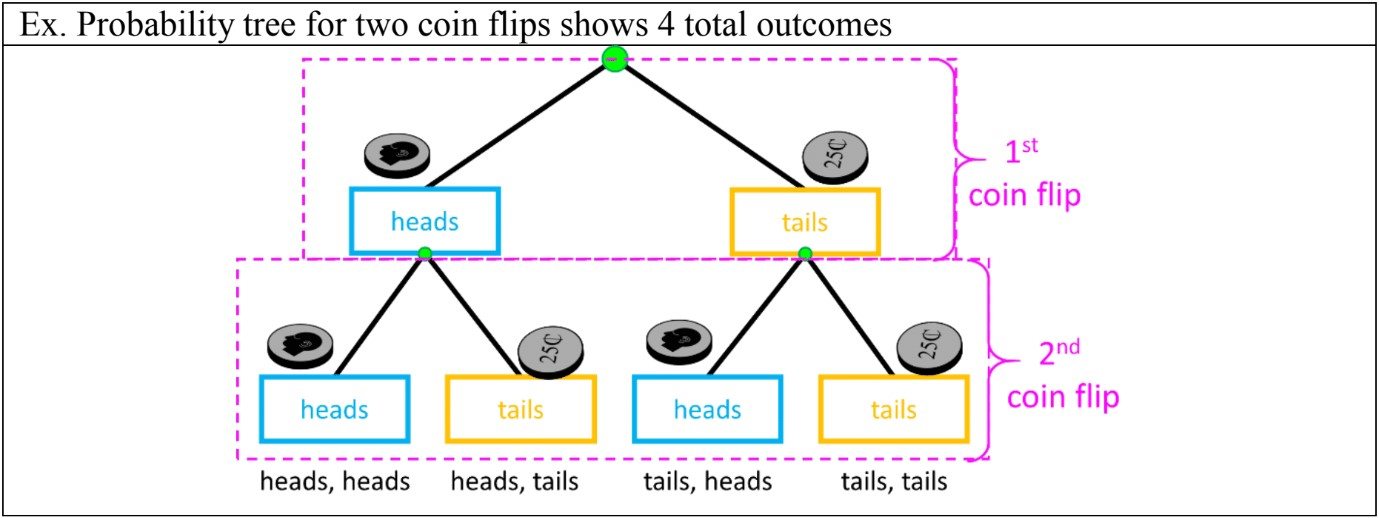• Another way to find all outcomes for compound events is by using an outcome table, crossing all outcomes for the first event in the rows, by all the outcomes for the second outcomes in the columns.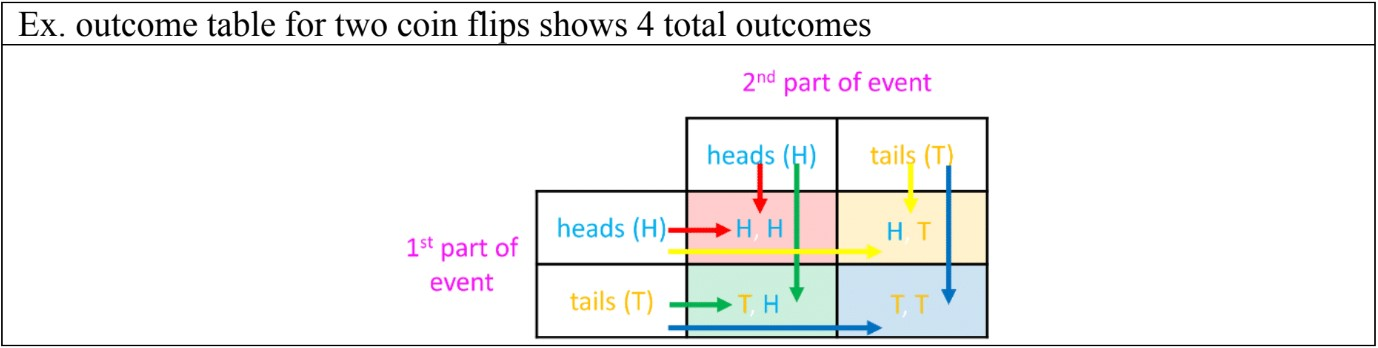• The total number of outcomes can be calculated:
total outcomes = (#outcomes 1st event) × (#outcomes 2nd event)

• The formula $P$ (event) = $\frac{number\,outcomes\,wanted} {total\,number\,possibilities}$ can also be used for compound probability
• Or, using fraction multiplication, compound probability for an event can also be found using the formula:

Compound Probability = $P$ (1st event) × $P$ (2nd event)